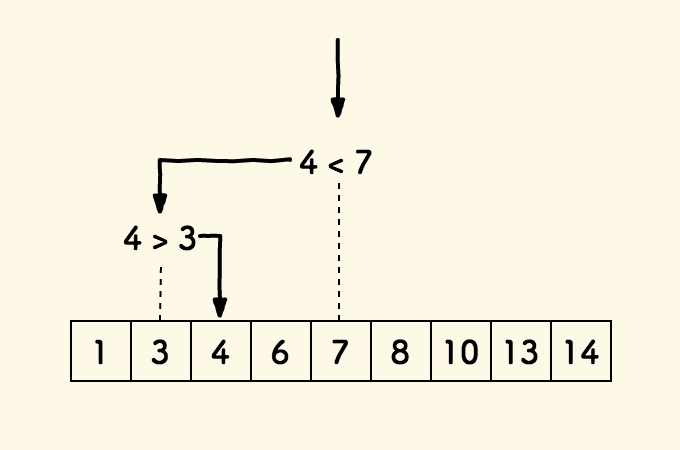# 搞定算法与数据结构 （01）：二分查找法的时间复杂度与空间复杂度分析

## 查找逻辑## 时间复杂度

F(n) = 1 + F(n/2)


F(n) = 1 + F(n/2)
= 1 + (1 + F(n/4)
= 2 + F(n/4)


F(n) = x + F(n/(2^x))


log_2^n + 1 = log_2^{10} * log_{10}^n+1 => O(log^n)

## 空间复杂度

1. 递归：由于每一次递归调用，都要额外申请栈空间，子级的栈空间是父级栈空间的一半，计算方法与上面计算时间复杂度相同，因此空间复杂度为 O(log^n)
2. 迭代：除了原数组本身会占用 n 个元素的内存空间外，其他不会再额外申请内存空间，因此空间复杂度为 O(n)